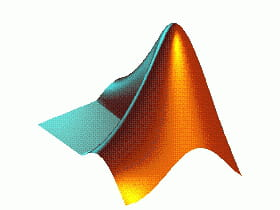0
Thanks

A few words of thanks would be greatly appreciated.

Concatenate vectors or matrices under Matlab

The operation to concatenate vectors or matrices under MATLAB is defined as a combination of the variables in a single vector or matrix. There are two types of concatenation operation - horizontal and vertical concatenation. For horizontal concatenation,two variables should have the same number of rows. In case of vertical concatenation,two variables should have the same number of columns. Every available variable cannot be concatenated. Concatenation operations with multiple variables in MATLAB software are possible as well, as one vector can be concatenated with the transpose of the same vector. It is possible to concatenate vectors or matrices under MATLAB in a logical way.Concatenation of variables in Matlab is defined as the combination of these variables in a single vector or matrix.

Example:

| 2 3 4 |
X =  | 1 2 5 |
| 0 2 7 |

With:
| 9 6 8 |
Y =  | 5 6 2 |
| 3 2 1 |

Concatenation of rows:
| 9 6 8 2 3 4 |
Result = | 1 2 5 5 6 2 |
| 0 2 7 3 2 1 |

Concatenation of columns:

| 2 3 4 |
| 1 2 5 |
| 0 2 7 |
Result =       | 9 6 8 |
| 5 6 2 |
| 3 2 1 |

Horizontal concatenation

Horizontal concatenation can be done using vectors, matrices or simple variables. The two variables (X and Y) must have the same number of rows. The code is as follows:

Result = [X Y]

Vertical concatenation

Vertical concatenation can be done using vectors, matrices or simple variables. The two variables (X and Y) must have the same number of columns. The code is as follows:

Result = [X, Y]

Further calculations

• Option 1

You can concatenate a row with a column, transposing a row as a column or vice versa. For example:

X = | 1 2 |

| 2 |
Y =  | 3 |
| 5 |

Using the command:

Result = [X Y]

Result = | 1 2 2 3 5 |

Using the command:

Result = [X ', Y]

| 1 |
| 2 |
Result =   | 2 |
| 3 |
| 5 |
• Option 2

You can extend this principle to cover multiple variables. For example. Using the following set of variable:
A = 1
B = 2
C = | 3 4 |
| 5 6 |
D = 7
E = | 8 9 |

The command:
Result = [[A;B] C;D E]

| 1 3 4 |
Result =  | 2 5 6 |
| 7 8 9 |
0
Thanks

A few words of thanks would be greatly appreciated.CCM is a leading international tech website. Our content is written in collaboration with IT experts, under the direction of Jeff Pillou, founder of CCM.net. CCM reaches more than 50 million unique visitors per month and is available in 11 languages.